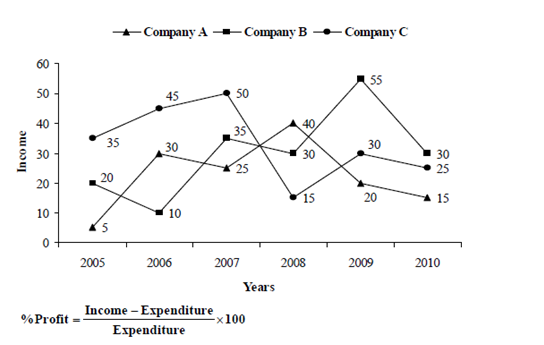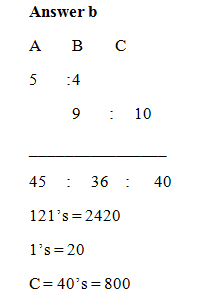# “20-20” Quantitative Aptitude | Crack SBI Clerk / IDBI Executive 2018 Day-45

Dear Friends, SBI Clerk 2018 Notification has been released we hope you all have started your preparation. Here we have started New Series of Practice Materials specially for SBI Clerk / IDBI Executive 2018 . Aspirants those who are preparing for the exams can use this “20-20” Quantitative Aptitude Questions.

[WpProQuiz 1527]

Click “Start Quiz” to attend these Questions and view Solutions

Daily Practice Test Schedule | Good Luck

 Topic Daily Publishing Time Daily News Papers & Editorials 8.00 AM Current Affairs Quiz 9.00 AM Logical Reasoning 10.00 AM Quantitative Aptitude “20-20” 11.00 AM Vocabulary (Based on The Hindu) 12.00 PM Static GK Quiz 1.00 PM English Language “20-20” 2.00 PM Banking Awareness Quiz 3.00 PM Reasoning Puzzles & Seating 4.00 PM Daily Current Affairs Updates 5.00 PM Data Interpretation / Application Sums (Topic Wise) 6.00 PM Reasoning Ability “20-20” 7.00 PM English Language (New Pattern Questions) 8.00 PM General / Financial Awareness Quiz 9.00 PM

Click Here for SBI Clerk Prelims and Mains 2018 – Full Length Mock Test

1. Rs.2420 were divided among A, B, C so that A: B = 5: 4 and B: C = 9: 10. Then what amount will C get?
1. Rs.720
2. Rs.800
3. Rs.900
4. Rs.960
5. Rs.700
1. The simple interest on a sum of money is 1/16 of the sum. If the number of years is equal to the rate percent per annum, then the rate percent per annum is
1. 3 1/3
2. 6 2/3
3. 2 ½
4. 7 ½
5. None of these
1. The speed of the current is 4 km/hr. A boat goes 10 km upstream and comes back to starting point in 80 min. What is the speed of the boat in still water? (In km/hr)
1. 10
2. 16
3. 14
4. 12
5. 8
1. The price of petrol is increased by 25%. By how much percent should a car owner should reduce his consumption of petrol so that the expenditure on petrol would not increase?
1. 25%
2. 50%
3. 30%
4. 20%
5. 35%
1. Out of 12 students studying in a class, 4 are from Maharashtra, 5 from Tamil Nadu and 3 from Goa. Four students are to be selected at random. What are the chances that at least one is from Tamil Nadu?
1. 92/99
2. 7/99
3. 16/77
4. 86/77
5. None of these

Direction (Q. 6-10): What will come in place of the question mark (?) in the following series?

1. 12, 13, 21, 48, 112, ?
1. 185
2. 237
3. 342
4. 174
5. None of these
1. 384, 65, 16, 9, 10,? , 25
1. 12
2. 18
3. 16
4. 14
5. None of these
1. 18, 9.5, 8.5, 14.25, 26.5, ?
1. 45.75
2. 51.75
3. 54.75
4. 68.75
5. None of these
1. 27, 43, 68, 118,? , 397
1. 178
2. 217
3. 318
4. 351
5. None of these
1. 31, 181, 899, 3589, ?
1. 10759
2. 7891
3. 6349
4. 9343
5. None of these

Directions (Q. 11 – 15): What approximate value should come in place of question mark (?) in the following questions?

1. 13873 + 133 % of 1605 – (7/5) of 4574 = ?
1. 6740
2. 9600
3. 8500
4. 12700
5. 7200
1. 5537 + 143 × 5.96 – 76.98 × 13 + 10.11 % of 1199 =?
1. 5520
2. 3480
3. 4730
4. 6790
5. 3670
1. 6499 + 3601 × 15.13 – 8790.21 =?
1. 48720
2. 32675
3. 51725
4. 42180
5. 58300
1. (5/7) of 7006 + (6/9) of 863 – 3 2/7 of 1763 =? – 526.31
1. 642
2. 311
3. 563
4. 432
5. 712
1. 32 × (?)3 + 17.082 + 601 = 1800
1. 5
2. 8
3. 3
4. 6
5. 4

Directions (Q. 16 – 20): Study the graph carefully to answer the questions that follow:

Income (in lakh Rs.) of three companies over the years:1. The average income per year for company A is approximately what percent of the average income per year for company C?
1. 71.6
2. 67.5
3. 148.15
4. 123.4
5. None of these
1. If the per cent profit of company A in the year 2006 was 50, what was its expenditure in that year?
1. 20 lakh
2. 25 lakh
3. 15 lakh
4. 12.5 Lakh
5. None of these
1. Income of Company A in the year 2006, 2008 and 2010 is approximately what percentage more or less than the Income of company B in the year 2005, 2007 and 2009?
1. 23 %
2. 35 %
3. 18 %
4. 29 %
5. None of these
1. What is the approximate percent increase in income of company C in year 2007 while comparing with the previous year?
1. 10%
2. 111%
3. 11%
4. 90%
5. None of these
1. If the expenditure of company B in 2007 was Rs.15 lakh, what was its percent profit in that year? (Rounded off to two digits after decimal)
1. 57.14%
2. 137.33%
3. 62.13%
4. 133.33%
5. None of these

1)S.I = (1 / 16) P

(1 / 16) P = (P × r × r) / 100 (Here n=r)

1 / 16 = r2 / 100

r2 =100/16

r = 10 / 4 = 2 ½ %

Let the speed of boat be x km/hr

According to the question, 10/(x – 4) + 10/(x + 4) = 80/60

=> 10 (x + 4 + x – 4) = 4/3(x2 – 16)

=> 15x = x2 – 16

=>x2 – 16 – 15x = 0

=> x = 16, -1

Therefore, the speed of boat in still water = 16km/hr

Reduction % in consumption

= [r/ (100 + r) x 100] %

= [25/ (100 + 25) x 100]

= 20%

Total probability= 12C4

Required probability= 1- none is from Tamil Nadu

= > 7C4

Required probability= 1- none is from Tamil Nadu

= > 1 – (7C4 / 12C4)

= > 1 – (7/99)

= > 92/99

Direction (Q. 6-10):

The pattern is, + (12 × 1), + (22 × 2), + (32 × 3), + (42 × 4), + (52 × 5)…

The pattern is, ÷6 +1, ÷5 +3, ÷4+5, ÷3 + 7, ÷2 +9, ÷1 + 11

The pattern is, *0.5 +0.5, *1 +1, *1.5 +1.5, *2 +2, *2.5+2.5….

The difference of difference is, 32, 52, 72, 92….

The pattern is, *6 -5, *5 -6, *4 -7, *3 -8,..

Directions (Q. 11 – 15):

13873 + (133/100) * 1600 – (7/5) * 4575 = x

X = 13873 +2128 – 6405

X = 9596

X= 9600(approx)

5537 + 143 × 6 – 77 × 13 + (10/100) × 1200 = x

X= 5537 + 858 – 1001 + 120

X = 5514 = 5520(approx)

6500 + 3601 × 15 – 8720 =x

X = 6500 + 54015 – 8790

X = 51725

(5/7) × 7007 + (6/9) × 864 – (23/7) × 1764 = x- 526

X = 5005 + 576 – 5796 + 526

X = 311

32 x3 + 289 + 601 = 1800

32 x3 = 1800 – 890

32 x3 = 910

X3 = 910/32 = 28

X= 3(approx)

Directions (Q. 16 – 20):

Required % = ([135/6]/[200/6]) x 100 = 67.5%

Let the expenditure was x lakhs

So, 50 = 30-x/x * 100

x = 20 lakhs

Income of Company A in the year 2006, 2008 and 2010

= > (30+40+15) = 85 lakhs

Income of company B in the year 2005, 2007 and 2009

= > (20+35+55) = 110 lakhs

Required % = ((110-85)/110)*100 = 22 8/11 % less = 23 %

Required % = (50-45)/45 x 100 = 11.11% ≈ 11%